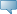# Lebanese American University WWW Information System

## Catalog Entries

Date
Sep 22, 2023Select the Course Number to get further detail on the course. Select the desired Schedule Type to find available classes for the course.

 MTH 301 - Linear Algebra This is an introductory course in linear algebra where students are exposed for the first time to a balance of computation, theory, and applications. Topics include the systems of linear equations, vector spaces Course Learning Outcomes: 1) Students will be able to solve linear systems using elementary row operation on matrices. Students will be able to solve applied problems using integrals. 2) Students will understand the concept of linear dependence and independence of vectors in a vector space and will be able to construct bases of vector spaces. 3) Students will have a complete understanding of linear transformations (definition, nullity, and rank) and will use matrices to represent such transformations). 4) Students will have a complete understanding of eigenvectors and eigenvalues and will use their knowledge for diagonalization. 3.000 Credit hours 3.000 Lecture hours Levels: Undergraduate Schedule Types: Lecture, Tutorial Computer Science & Mathematics Department